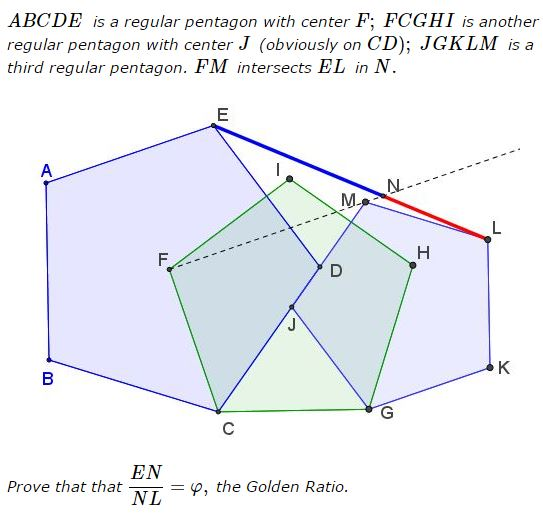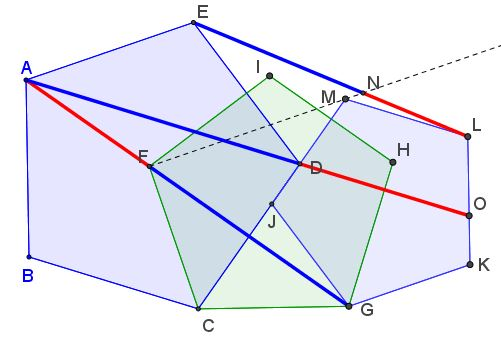# Golden Ratio in Three Regular Pentagons

### Problem### Solution

Solutions are welcome.

### Extra

The configuration of three regular pentagons proved beckoning and promising. After a misstep that used only two pentagons, $\displaystyle \frac{FG}{AF}=\varphi,\,$ another occurrence of the Golden Ratio, authentically in three pentagons has popped up:

Let $AD\,$ intersect $LK\,$ in $O.\,$Then $\displaystyle \frac{AD}{DO}=\varphi,\,$ the Golden Ratio.

### Acknowledgment

The problem has been kindly posted on the CutTheKnotMath facebook page by Tran Quang Hung.